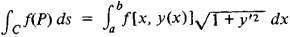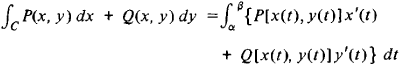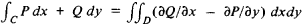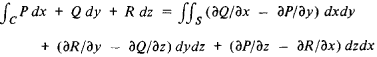Line Integral

(redirected from Tangential line integral)

line integral

[′līn ¦int·ə·grəl]
(mathematics)
For a curve in a vector space defined byx=x(t), and a vector functionVdefined on this curve, the line integral ofValong the curve is the integral over t of the scalar product ofV[x(t)] and d x/ dt ; this is written ∫V· d x.
For a curve which is defined by x = x (t), y = y (t), and a scalar function ƒ depending on x and y, the line integral of ƒ along the curve is the integral over t of ƒ[x (t), y (t)] · √(dx / dt)2+ (dy / dt)2); this is written ∫ ƒ ds, where ds = √(dx)2+ (dy)2) is an infinitesimal element of length along the curve.
For a curve in the complex plane defined by z = z (t), and a function ƒ depending on z, the line integral of ƒ along the curve is the integral over t of ƒ[z (t)] (dz / dt); this is written ∫ ƒ dz.
McGraw-Hill Dictionary of Scientific & Technical Terms, 6E, Copyright © 2003 by The McGraw-Hill Companies, Inc.
The following article is from The Great Soviet Encyclopedia (1979). It might be outdated or ideologically biased.

Line Integral

an integral taken along some curve in the plane or in space. We distinguish line integrals of the first kind and line integrals of the second kind. A line integral of the first kind arises, for example, in problems involving the calculation of the mass of a curve of variable density and is denoted by

Cf (P)ds

where C is the given curve, ds is the differential of its arc, and f(P) is the function of a point on the curve and is the limit of the corresponding integral sums. In the case of a plane curve C given by the equation y = y(x), a line integral of the first kind reduces to an ordinary integral. Specifically,A line integral of the second kind arises, for example, in connection with the work of a force field. In the case of a plane curve C the integral takes the form

CP(x,y)dx + Q(x,y)dy

and is also the limit of the corresponding integral sums. A line integral of the second kind can be expressed as an ordinary integral. Specifically,wherex = x(t) and y = y(t) for α ≤ t ≤ β, is the parametric equation of the curve C. Its connection with a line integral of the first kind is given by the equality

CP(x,y)dx + Q (x,y)dy = ∫C {P cosα + Q sin α} ds

Here, α is the angle between the Ox axis and the tangent to the curve pointing in the direction of the increasing arc length.

A line integral of the second kind in space is defined similarly. (SeeVECTOR CALCULUS for a treatment of line integrals of the second kind from the standpoint of vectors.)

Suppose D is some region and C is its boundary. Under certain conditions, the line integral along the curve C and the double integral over the region D (see) are connected by the relationSimilarly, the line integral and the surface integral are connected by the relationLine integrals are of great importance in the theory of functions of a complex variable. They are widely used in various branches of mechanics, physics, and engineering.